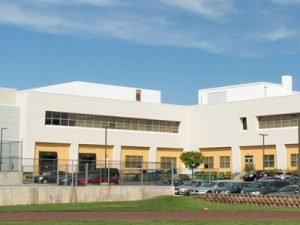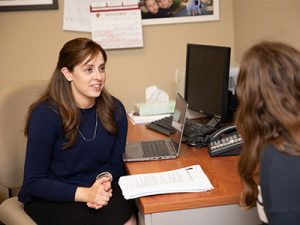Generic selectors
Exact matches only
Search in title
Search in content
Post Type Selectors
• Home
••••• Get To Know Us
• Calendar

## Math

The Mathematics Department at Frisch offers a progressive and innovative college preparatory program. Students are not only taught a wide range of topics in math, but also how to think and view the world from a quantitative perspective. Using the most progressive technology, real time assessment, blended learning, group work, and frontal teaching, Frisch students receive a rich and sophisticated math education which prepares them for standardized tests and future college level studies in mathematics. Multiple tracks of every math course are offered to meet the needs of our students’ different learning styles.

### Geometry

This course is taught at various levels and is a comprehensive college-prep year of Geometry. Freshmen who demonstrate a mastery of Algebra One on the Frisch math placement test and who have done exceptionally well on prior standardized math tests are placed in  Honors Geometry. Theorems and postulates about lines, angles, triangles, polygons, and circles are explored using various software programs such as Geogebra and Smartboard Math tools. Additionally, the topics of congruence, similarity, volume, surface area, special right triangles and right triangle trigonometry, as well as the exercise of writing formal two column statement-reason proofs, are taught. Students who are mathematically precocious and enter high school having already mastered the curriculum of even our most advanced 9th grade classes are placed in higher level math classes or work independently with members of Frisch’s math department.

The course is a bridge from the concrete to the abstract study of mathematics, as students progress through the Algebra I curriculum. Topics include factoring, simplifying expressions, graphing functions and relations, ratios and proportions, evaluating and solving equations and inequalities, and simplifying radicals. Real world applications are presented within the course content. Lessons require an understanding of Geometry topics studied in their previous course to show connections between the two branches of mathematics.

### Honors level Algebra II

In this course, students learn more advanced topics in Algebra and how to execute the more complex algebraic manipulations involved with linear equations, inequalities, exponents, rational functions, quadratics, variation, and polynomials. Heavy emphasis is placed on learning to graph functions and understand their various characteristics such as domain, range, zeros, intercepts, and end behavior. Additionally, students gain experience with analyzing graphs using both the online graphing calculator Desmos and the TI-84 graphing calculator.

(see the description above)

### Precalculus (Honors)

The Precalculus course covers advance theorems of polynomials, exponential and logarithmic functions, right triangle and unit circle based trigonometry, sequences/ series and polar coordinates. Limits and derivatives are introduced and a TI- 84 graphing calculator and Desmos are used extensively.

## Junior Electives

(In addition to Precalculus or Algebra II required courses)

### Survey of Mathematics

This survey course is geared towards preparing students for standardized exams and exposing students to various topics within math. Within the specific framework of the SAT, all mathematical concepts under the “Heart of Algebra,” “Problem Solving and Data Analysis,” “Passport to Advanced Math,” and “Additional Topics in Math” are covered. Within the framework of the ACT, all concepts under the “Pre-Algebra,” “Elementary Algebra,” “Intermediate Algebra,” “Coordinate Geometry,” “Plane Geometry,” and “Trigonometry” are covered. Standardized questions are presented and approaches to the questions are discussed. Students are mentored not only in the actual mathematics, but also in specific test-taking strategies. Practice exams that simulate actual testing conditions are administered to assist students in preparing for standardized tests.

### Finite Math Workshop

Finite Math has several major themes: systematic counting, using discrete mathematical models, applying mathematical processes to real-world scenarios, and collecting and organizing information. Some topics include combinatorics, matrix models, probability, expected value, symbolic logic, logarithmic  functions, and exponential growth and decay. Students will also study descriptive and inferential statistics, (which includes representing data visually, calculating measures of central tendency, and computing standard  deviation and z-scores). Students are assessed by open-note tests, projects, and oral presentations. With an invigorating emphasis on real world applications, the course incorporates individual and small-group problem solving with a focus on meaningful application of mathematics. This course is not for those students who plan to take Finite Math as their senior math requirement.

### Financial Literacy

In this Algebra-based course, students develop  skills and strategies that promote personal and financial responsibility related to financial planning, savings, investment, and charitable giving in the global economy.

Students learn about taxes and tax deductions and why fees for various benefits (e.g., medical benefits) are taken out of pay, as well as information about investment options that assist with financial planning.

### Calculus 1 (Honors)

Topics in differential and integral calculus are covered, as students gain a deeper understanding of the behavior of functions through their study of limits and the derivative. In addition to learning techniques of differentiation, they also explore applications such as optimization and related rates problems. The integral is introduced through discussion of the Riemann Sum and the antiderivative.

In the second half of the course, students focus on applications of the Fundamental Theorem of Calculus, techniques of integration, area between curves, and volumes of solids of revolution. Students in this course are prepared to take the College Board Advanced Placement Test in AB Calculus.

### Calculus 2 (Honors)

Topics in differential and integral calculus are covered, as students build on their existing knowledge of limits and differentiation in order to explore the integral and its uses. In addition to applications such as volume of solids of revolution, students explore higher-level integration techniques such as integration by parts and the use of trigonometric integrals. In the second half of the course, students focus on differential equations, the calculus of parametric and polar equations, and infinite series. Students in this course are prepared to take the College Board Advanced Placement Test in BC Calculus.

### Statistics

The broad themes of this course are exploring data, sampling and experimentation, anticipating patterns and statistical inference. Statistics is taught on the introductory level, as well as the advanced level, which prepares students for the Advanced Placement exam.

### PreCalculus

A rigorous college preparatory course, students gain the knowledge and skills necessary for the future study of Calculus in college. Topics covered include polynomials, exponential and logarithmic functions, trigonometry and sequences and series. The TI- 84 graphing calculator and DESMOS will be used extensively. Since the course is a continuation and culmination of topics from Algebra 1 and Algebra 2, this course is designed for students with a strong academic record in mathematics.

### Applied Math

Students apply the concepts and methods of finite mathematics to model and explore a variety of practical situations. Themes include systematic counting, using discrete mathematical models, applying mathematical processes to real-world scenarios, and collecting and organizing information. Topics covered include combinatorics, matrix models, probability, expected value, symbolic logic, logarithmic functions, and exponential growth and decay. Students also study descriptive and inferential statistics, which includes representing data visually, calculating measures of central tendency, and computing standard deviation and z-scores.